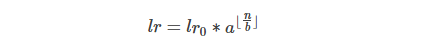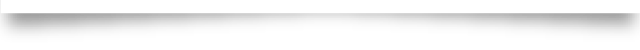# 【图像分类】使用经典模型进行图像分类

2018/03/20 22:09

3.19：【图像分类】

3.20：【图像分类】

3.21：【目标检测】

SSD目标检测

3.22：【场景文字识别】

|1. 训练模型

初始化

import gzip

import argparse

import paddle.v2.dataset.flowers as flowers

import vgg

import resnet

import alexnet

import inception_v4

import inception_resnet_v2

import xception

定义参数和输入

# Use 3 * 331 * 331 or 3 * 299 * 299 for DATA_DIM in Inception-ResNet-v2.

DATA_DIM = 3 * 224 * 224

CLASS_DIM = 102

BATCH_SIZE = 128

获得所用模型

• 使用AlexNet模型

out = alexnet.alexnet(image, class_dim=CLASS_DIM)

• 使用VGG模型

out = vgg.vgg16(image, class_dim=CLASS_DIM)

input=out1, label=lbl, coeff=0.3)

input=out2, label=lbl, coeff=0.3)

extra_layers = [loss1, loss2]

• 使用ResNet模型

ResNet模型可以通过下面的代码获取：

out = resnet.resnet_imagenet(image, class_dim=CLASS_DIM)

• 使用Inception-v4模型

Inception-v4模型可以通过下面的代码获取, 本例中使用的模型输入大小为3 * 224 * 224 (原文献中使用的输入大小为3 * 299 * 299)：

out = inception_v4.inception_v4(image, class_dim=CLASS_DIM)

• 使用Inception-ResNet-v2模型

out = inception_resnet_v2.inception_resnet_v2(

image, class_dim=CLASS_DIM, dropout_rate=0.5, size=DATA_DIM)

• 使用Xception模型

Xception模型可以通过下面的代码获取：

out = xception.xception(image, class_dim=CLASS_DIM)

定义损失函数

cost = paddle.layer.classification_cost(input=out, label=lbl)

创建参数和优化方法

# Create parameters

# Create optimizer

momentum=0.9,

BATCH_SIZE),

learning_rate=0.001 / BATCH_SIZE,

learning_rate_decay_a=0.1,

learning_rate_decay_b=128000 * 35,

learning_rate_schedule="discexp", )定义数据读取

flowers.train(),

buf_size=1000),

batch_size=BATCH_SIZE)

flowers.valid(),

batch_size=BATCH_SIZE)

dataset_100/train_images/n03982430_23191.jpeg    1

dataset_100/train_images/n04461696_23653.jpeg    7

dataset_100/train_images/n02441942_3170.jpeg 8

dataset_100/train_images/n03733281_31716.jpeg    2

dataset_100/train_images/n03424325_240.jpeg  0

dataset_100/train_images/n02643566_75.jpeg   8

buf_size=1000),

batch_size=BATCH_SIZE)

batch_size=BATCH_SIZE)

定义事件处理程序

# End batch and end pass event handler

def event_handler(event):

if event.batch_id % 1 == 0:

print "\nPass %d, Batch %d, Cost %f, %s" % (

event.pass_id, event.batch_id, event.cost, event.metrics)

with gzip.open('params_pass_%d.tar.gz' % event.pass_id, 'w') as f:

parameters.to_tar(f)

print "\nTest with Pass %d, %s" % (event.pass_id, result.metrics)

定义训练方法

# Create trainer

cost=cost,

parameters=parameters,

update_equation=optimizer)

# Create trainer

cost=cost,

parameters=parameters,

update_equation=optimizer,

extra_layers=extra_layers)

开始训练

trainer.train(

|2.应用模型

with gzip.open('params_pass_10.tar.gz', 'r') as f:

file_list = [line.strip() for line in open(image_list_file)]

.flatten().astype('float32'), )

for image_file in file_list]

output_layer=out, parameters=parameters, input=test_data)

lab = np.argsort(-probs)

for file_name, result in zip(file_list, lab):

print "Label of %s is: %d" % (file_name, result)

|3. 使用预训练模型

end

*原创贴，版权所有，未经许可，禁止转载

*欢迎在留言区分享您的观点

*为了方便大家问题的跟进解决，我们采用Github Issue来采集信息和追踪进度。大家遇到问题请搜索Github Issue，问题未解决请优先在Github Issue上提问，有助于问题的积累和沉淀### 作者的其它热门文章

0
0 收藏

0 评论
0 收藏
0